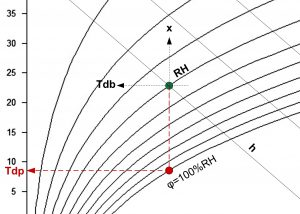# Dew point temperature

Dew point temperature: Tdp [°C]

It is the temperature detectable for a given state of wet air at saturation for specific absolute humidity x. In the h-x diagram we draw a vertical line along a constant absolute humidity (x = constant) down to the saturation curve (RH = 100%) and at this point the isotherm at the intersection indicates the dew point temperature Tdp.

Tdp=  (237,7 * ((17,271 * Tdb) / (237,7 + Tdb) + LN(RH * 0,01))) / (17,271 – ((17,271 * Tdb) / (237,7 + Tdb) + LN(RH * 0,01)))